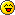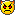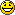# University is all fun and games!##University is all fun and games!

I wrote this for a thread in a physics forum, as I need help with part of my fluid dynamics assignment - but thought I'd post it here incase you get the idea that I just sit around playing WW2OL all day without a care in the world.1. The problem statement, all variables and given/known data

A Farmer builds a water storage tank 12m above ground, as shown below:The water is fed via a 200m long, 125mm diameter pipe to a field for irrigation purposes. The pipe has a friction factor of 0.008. Using the K data below, show that the water flow rate (kg/s) in the pipe is approximately 26kg/s

Data:

K Factors: Tank Exit 0.5, Bends 0.9 (x2), Valve 1.0 (Total: 3.3)
Water Density: 1000kg/m3

2. Relevant equations

Bernoulli's Equation: (P_1/ρ*g) + (V_1^/2*g) + z1 = (P_2/ρ*g) + (V_2^/2*g) + z2
Darcy Formula: H_f = 4*f*(L/D)*(V^/2*g)
Moody Diagram

3. The attempt at a solution

Right then, I've been asked to find the water flow rate, which I'm presuming means the mass flow rate (kg/s) - so in order to find that I need the volume flow rate, and in order to find that I need the velocity

I haven't been given the velocity in the original problem, however I think I need to use the Bernoulli Equation in order to find the velocity.

I've made a few assumptions with the Bernoulli Equation, which are that the pressure at point 1 (the water tank) is atmospheric (101325pa) - because on the picture the tank looks to be open to atmosphere, I've also assumed that the pressure at point 2 (the end of the pipe) is also atmospheric (101325pa) because the pipe is leading to a field.

As well as this I've assumed that the velocity at point 1 is approximately 0m/s (because the water is going from a large tank into a small pipe)

So at the moment, my Bernoulli equation looks like:

(101325 / (1000 x 9.81)) + (0^ / (2 x 9.81)) + 12 = (101325 / (1000 x 9.81)) + (V_2^ / (2 x 9.81)) + 0

However I understand that this equation isn't full, as I also have to take into account the friction (head loss), which would change the Bernoulli equation into:

(P_1/ρ*g) + (V_1^/2*g) + z1 = (P_2/ρ*g) + (V_2^/2*g) + z2 + h_f

So in order to accurately find the velocity using the Bernoulli equation, I have to also take into account the Head loss?

But the Darcy Formula is: H_f = 4*f*(L/D)*(V^/2*g)

So in order to use the Darcy Formula I have to know the velocity - but I don't know the velocity (as that's what I'm trying to find)? - Unless there's a way of finding the head loss without knowing the velocity?

Which somewhat puts me at a dead end.

Another route I was thinking of using to find the velocity was to use the Moody Diagram to try to find the Reynolds number, then using Re=(ρ*V*D)/μ to find the velocity (as I know all the other values.)

However the only other Moody diagram value I know is the Friction Factor of 0.008, and I'm unable to find the Relative roughness (k/D) as the problem doesn't state what sort of pipe is being used (so I can't find the roughness.)

Bloody Aeronautical Technology course my arse! Seem to spend all the time trying to help some bloody farmer funnel crap down pipes!Crap like this just makes me wish I'd become a Binman...

Incidentally, if any of you chaps happen to know how to do this, I'd be awfully grateful.I did manage to make something that looked like one of the exhausts from a Spitfire Mk.I/II in CAD today though which was fun, it wasn't designed to look like one of the exhausts from a Spitfire Mk.I/II mind, it just turned out that way._________________"I look forward confidently to the exploits of our Fighter Pilots - these splendid men, this brilliant youth, who will have the glory of saving their native land, their island home, and all they love, from the most deadly of all attacks."

- Winston ChurchillMJDixon

Posts : 5741
Join date : 2010-01-11
Age : 31
Location : Great Britain

##Re: University is all fun and games!

out of interest what CAD package are you tinkering with?speedy77

Posts : 1633
Join date : 2009-09-02
Age : 42
Location : Dorset

##Re: University is all fun and games!

Im with Speedy on this. Also I believe Bernoulli has a lot to answer for. The diagram looks quite cheerful btw :-)Dezey

Posts : 596
Join date : 2009-09-09

##Re: University is all fun and games!

Using PTC Creo Elements (Student Version) for CADage, used to be called Pro/Engineer Wildfire. If you're lucky I might post some amazing screenshots later of a teapot!111one

As for the physics thing, apparantly I just have to keep guessing the velocity and putting it into the equation until it fits.(i.e I know all the stuff for the first point in the system, so I need to keep putting a velocity into the second one until the total matches the first one (as Bernoulli's Equation says that the total of everything at point 1 has to be the same as the total of everything at point 2.)

Doesn't sound very scientific if you ask me._________________"I look forward confidently to the exploits of our Fighter Pilots - these splendid men, this brilliant youth, who will have the glory of saving their native land, their island home, and all they love, from the most deadly of all attacks."

- Winston ChurchillMJDixon

Posts : 5741
Join date : 2010-01-11
Age : 31
Location : Great Britain

##Re: University is all fun and games!